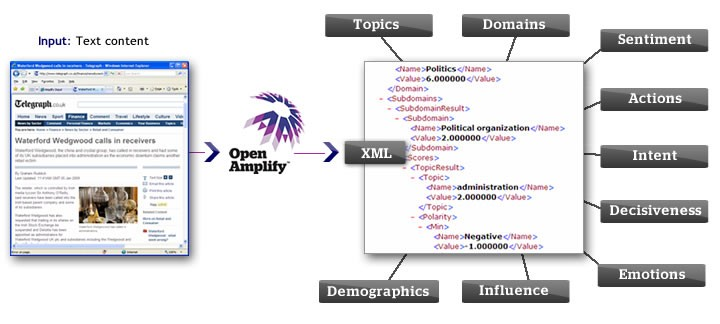# What is a Term-document Matrix?

A term-document matrix is an important representation for text analytics.

Each row of the matrix is a document vector, with one column for every term in the entire corpus.

Naturally, some documents may not contain a given term, so this matrix is sparse. The value in each cell of the matrix is the term frequency. (This value is often a weighted term frequency, typically using tf-idf – term frequency-inverse document frequency.)

## Similarity

With the term-document matrix, you can compute the similarity of documents. Just multiply the matrix with its own Linear Algebra - Matrix S = DDT, and you have an (unnormalized) measure of similarity.

The result is a square document-document matrix, where each cell represents the similarity. Here, similarity is pretty simple: if two documents both contain a term, then the score goes up by the product of the two term frequencies. This score is equivalent to the dot product of the two document vectors.

``````Matrix D                                 The transpose (D)
term1   term2   term3                   doc1     doc2     doc3
doc1         3       0       1           term1      3        0        2
doc2         0       1       1           term2      0        1        1
doc3         2       1       0           term3      1        1        0
```
```
``````SELECT
Matrix.row_num,
Transpose.col_num,
SUM(Matrix.value*Transpose.value)
FROM
(SELECT docid AS row_num, term AS col_num, COUNT AS value FROM frequency
) Matrix,
(SELECT term AS row_num, docid AS col_num, COUNT AS value FROM frequency
) Transpose
WHERE
Matrix.col_num = Transpose.row_num AND
Matrix.row_num < Transpose.col_num
GROUP BY
Matrix.row_num,
Transpose.col_num;```
```

You don't need to compute the similarity of both (doc1, doc2) and (doc2, doc1) – they are the same, since similarity is symmetric. You can avoid this wasted work by adding a condition of the form Matrix.docid < Tranpose.docid to the query.

To normalize this score to the range 0-1 and to account for relative term frequencies, the cosine similarity is perhaps more useful.

### Primitive search capabilities

Add a fictive document that contains the search words and compute the similarity matrix only for this document

``````SELECT * FROM frequency
UNION
SELECT 'search' as docid, 'washington' as term, 1 as count
UNION
SELECT 'search' as docid, 'taxes' as term, 1 as count
UNION
SELECT 'search' as docid, 'treasury' as term, 1 as count```
```

Discover MoreNatural Language - Text Modeling

denormalizing the schema to have each word appear only once with a list of occurrences per word, i.e. word, list . It allows for aggressive delta-compression of the list (typically called a ), which is...